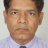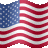# Combining Pullback and CCI scan

N

#### Nick

##### Active member
2019 Donor
VIP
Hi @BenTen, i would like to create a simple scan for stocks that have pullback to 30Day Moving Average and it is also oversold using CCI (-100). This is something i tried but was unable to get scan results that satisfy both conditions. It seems to be able to fulfill the Moving Average condition but not the CCI.... Is there something wrong with the code? Thank you

declare lower;

input bars = 30;
def ma = SimpleMovingAvg(close, bars);
def s = ma * 0.01;
def scan = AbsValue(close - ma) <= s;

################################################

def CCI = -100;

################################################

plot Signal = scan and CCI -100 ;#### BenTen

Staff
VIP
So let me get the condition straight, you want the stock to be crossing the 30 day moving average while CCI is at oversold?

N

#### Nick

##### Active member
2019 Donor
VIP
So let me get the condition straight, you want the stock to be crossing the 30 day moving average while CCI is at oversold?
@BenTen Yes, i thought about this part too, what i wanted is the stock close price is at least touch 1% from the 30D Moving Average. The reason why i set this 1% rule is avoid having too many stocks that their prices are far away from 30 day moving average. Not too sure if this condition is valid. If it doesn't, i am ok to use when price cross below 30D moving average as the condition. Thank you very much.#### BenTen

Staff
VIP
@Nick Here is how you can set it up:`close crosses SimpleMovingAvg("length" = 30)."SMA" and CCI()."CCI" is greater than or equal to 100`

N

#### Nick

##### Active member
2019 Donor
VIP
@BenTen Thank You. Didn't know it can be so simple. May i know if there are any differences with the scan results if the scan is done with separate the conditions vs one scan code with both codes combined as a single scan?#### BenTen

Staff
VIP
@Nick You'll most likely get more results if you don't filter them out using both conditions at the same time.

C

#### camerdr

##### New member
VIP
@BenTen, would you please help me with this script? I want it to plot a SMA along with CCI in the lower studies and produce an arrow when there is a cross. In my script below, the CCI is fine but the SMA is just a straight line all across.

Thanks

Code:
``````declare lower;

input CCI_length = 14;
input lookback = 2;

#MvgAvg

input averageType = AverageType.SIMPLE;
input MvgAvg_length = 20;
input MvgAvg_price = close;
plot SMA = MovingAverage(averageType, MvgAvg_price, MvgAvg_length);

def CCI_price = close + low + high;
def linDev = lindev(CCI_price, CCI_length);
plot CCI = if linDev == 0 then 0 else (CCI_price - Average(CCI_price, CCI_length)) / linDev / 0.015;

def UpSignal = CCI crosses above SMA;
def DownSignal = CCI crosses below SMA;

SMA.SetDefaultColor(GetColor(1));
CCI.SetDefaultColor(GetColor(2));

plot ArrowUp = if CCI crosses above SMA
then low
else double.nan;
ArrowUP.SetPaintingStrategy(PaintingStrategy.Arrow_UP);
ArrowUP.SetLineWeight(3);
ArrowUP.SetDefaultColor(Color.Green);
plot ArrowDN = if CCI crosses below SMA
then high
else double.nan;
ArrowDN.SetPaintingStrategy(PaintingStrategy.Arrow_DOWN);
ArrowDN.SetLineWeight(3);
ArrowDN.SetDefaultColor(Color.Red);``````#### BenTen

Staff
VIP
@camerdr Not possible. This is like trying to mix water with oilC

#### camerdr

##### New member
VIP
@camerdr Not possible. This is like trying to mix water with oil@BenTen, this is the TradingView script. How can one convert it from pinescript?

Code:
``````study(title="CCIEMA", shorttitle="CCI+EMA by perrito Blacky")
length1 = input(20, minval=1, title="CCI")
ma_length = input(13, minval=1, title="EMA")
src = input(close, title="Source")
ma1 = sma(src, length1)
cci1 = (src - ma1) / (0.015 * dev(src, length1))
//plot(cci1, color=blue, linewidth=2)
plot(cci1, linewidth=2)
//plot(ema(cci1, ma_length), color=green, linewidth=2)
plot(ema(cci1, ma_length), linewidth=2)

plotColour = (cci1 > ema(cci1, ma_length)) ? green : red
plotCCIEMA = plot(series=cci1, color=plotColour, linewidth=2)
//plotHoriz  = plot(series=0, color=blue, style=circles)
//fill(plot1=plotCCIEMA, plot2=plotHoriz, color=blue, transp=90)

band0 = hline(200, color=red)
band1 = hline(100, color=blue)
band2 = hline(0,title="zero")
band3 = hline(-100, color=blue)
band4 = hline(-200, color=red)
fill(band1, band3, color=blue, transp=95)``````#### BenTen

Staff
VIP
@camerdr Try this:

Code:
``````# CCI + EMA
# Rough conversion by BenTen at UseThinkScript.com

declare lower;

input length1 = 20;
input ma_length = 13;
input src = close;

def ma1 = simpleMovingAvg(src, length1);
def cci1 = (src - ma1) / (0.015 * stdev(src, length1));

plot cci_line = cci1;
plot cci_ema = expAverage(cci1, ma_length);

plot band0 = 200;
plot band1 = 100;
plot band2 = 0;
plot band3 = -100;
plot band4 = -200;``````Exploring Scan Combining Unusual volume and near 52 weeks high ... Playground 26Combining Lower Indicators on One Window? Questions 8